# 两轮平衡小车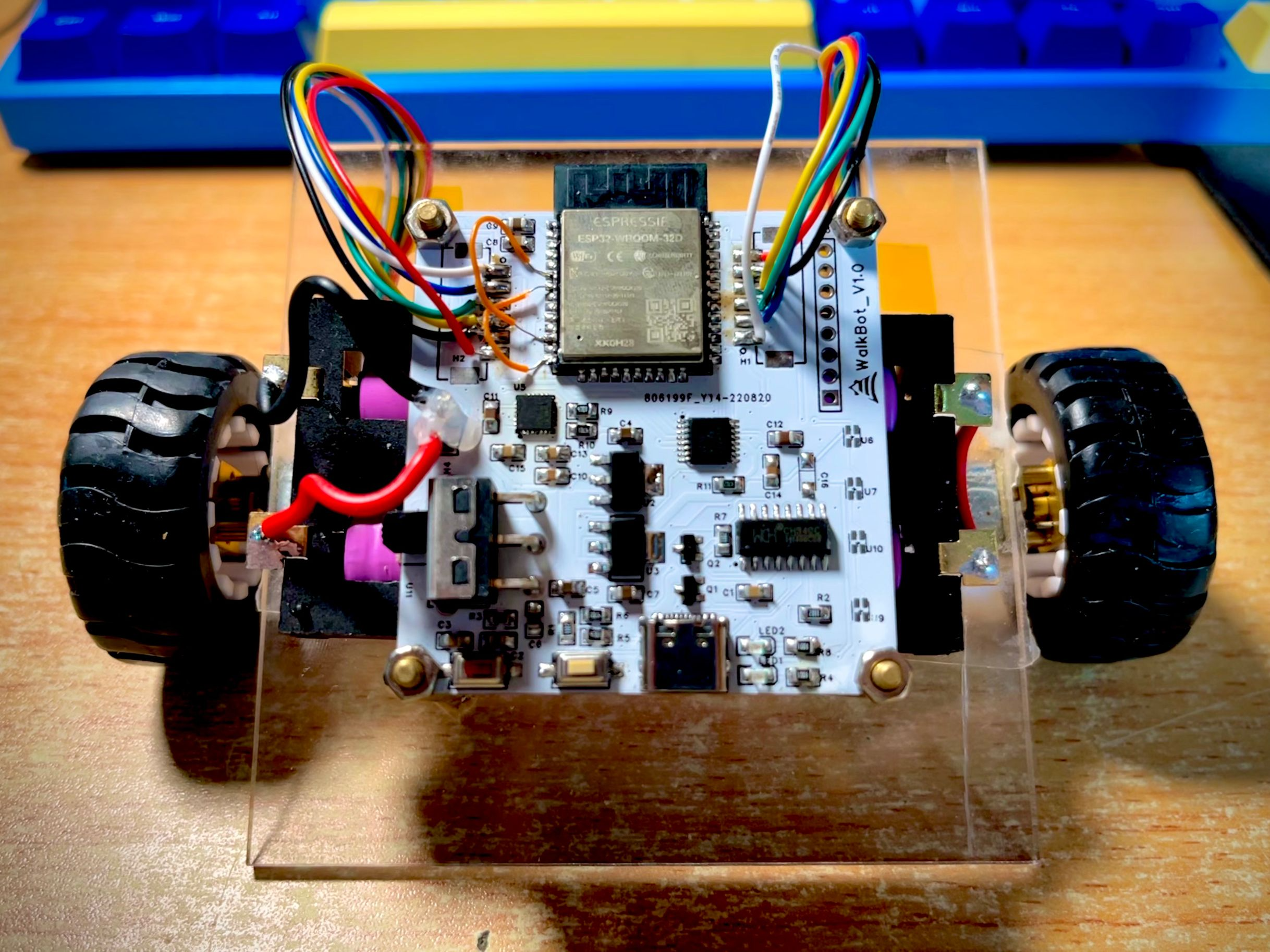## 初步构思

• 首先既然是平衡车，那么一定要有两个轮子，当然还有通过转轴和轮子连接的电机。
• 而要实现对电机的控制，需要电机控制模块，也就是我们整个系统的控制板。控制板上还要有运动传感器，来检测当前的运动状态。
• 还需要有电池作为整个系统的电力来源，连接到控制板上。
• 仅凭一块控制板是难以同时放下电池并固定好电机的，所以我们还需要一层亚力克底板，作为系统的加固和支撑，亚克力材质也方便我们打孔固定。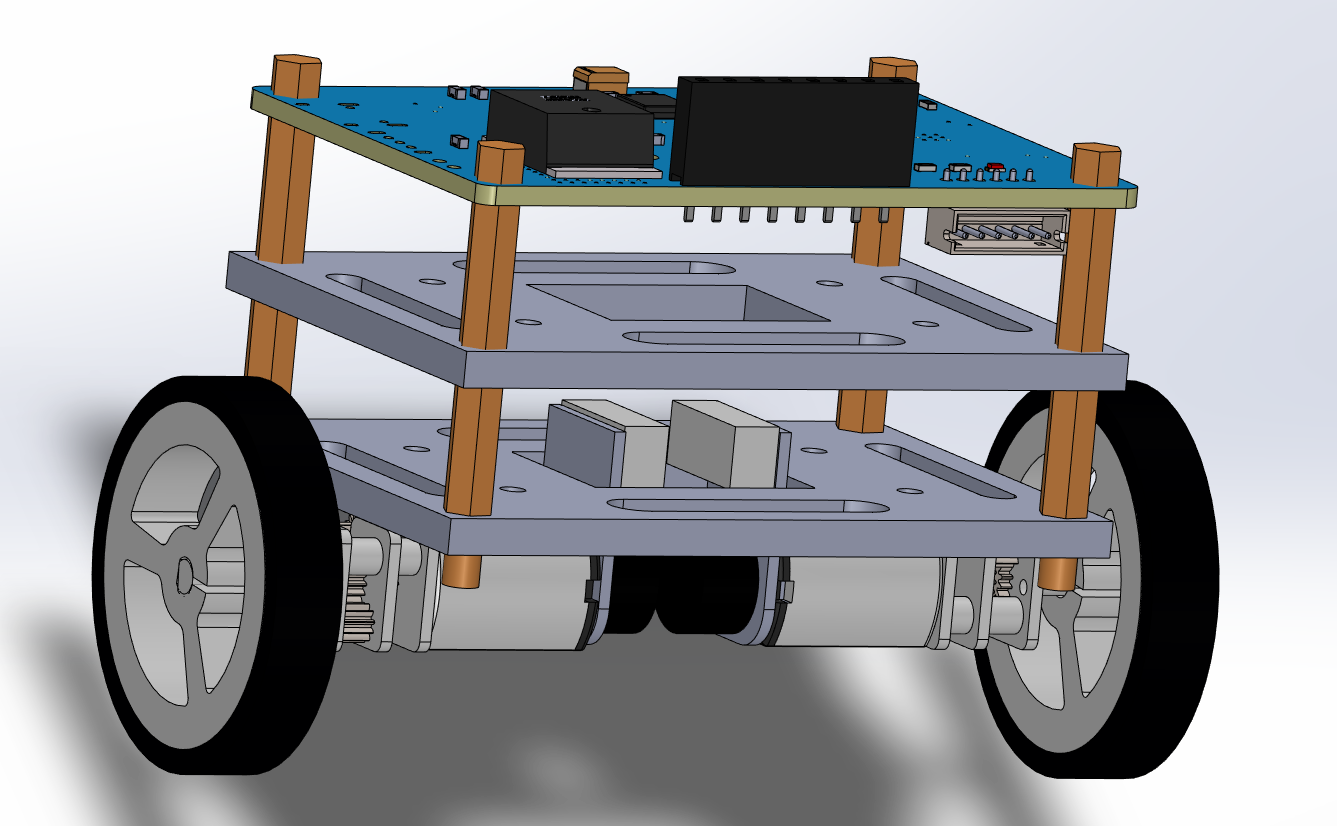## 选型与设计

### 电机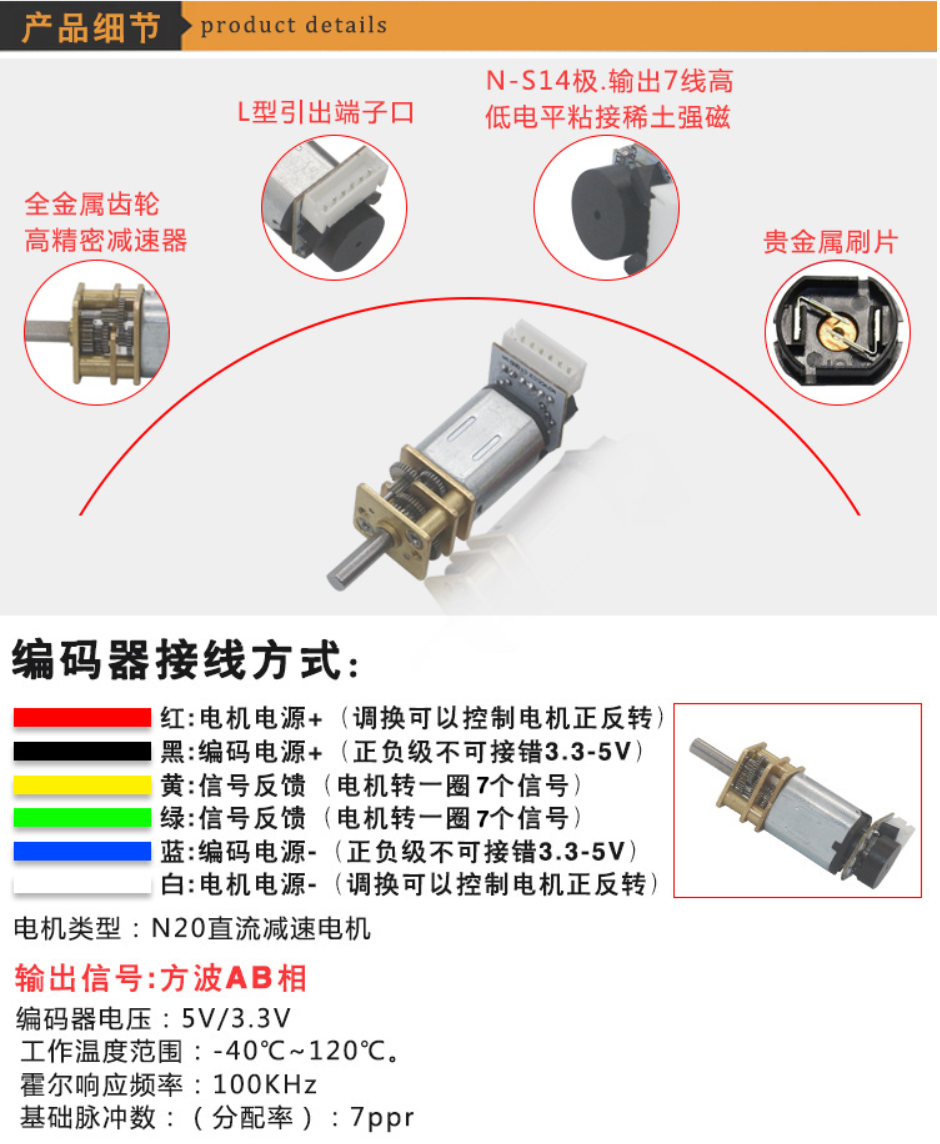DRV8833电机驱动芯片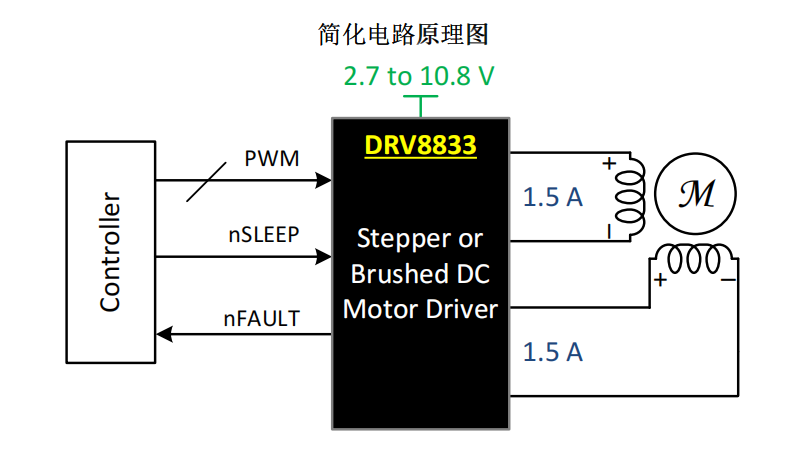MCU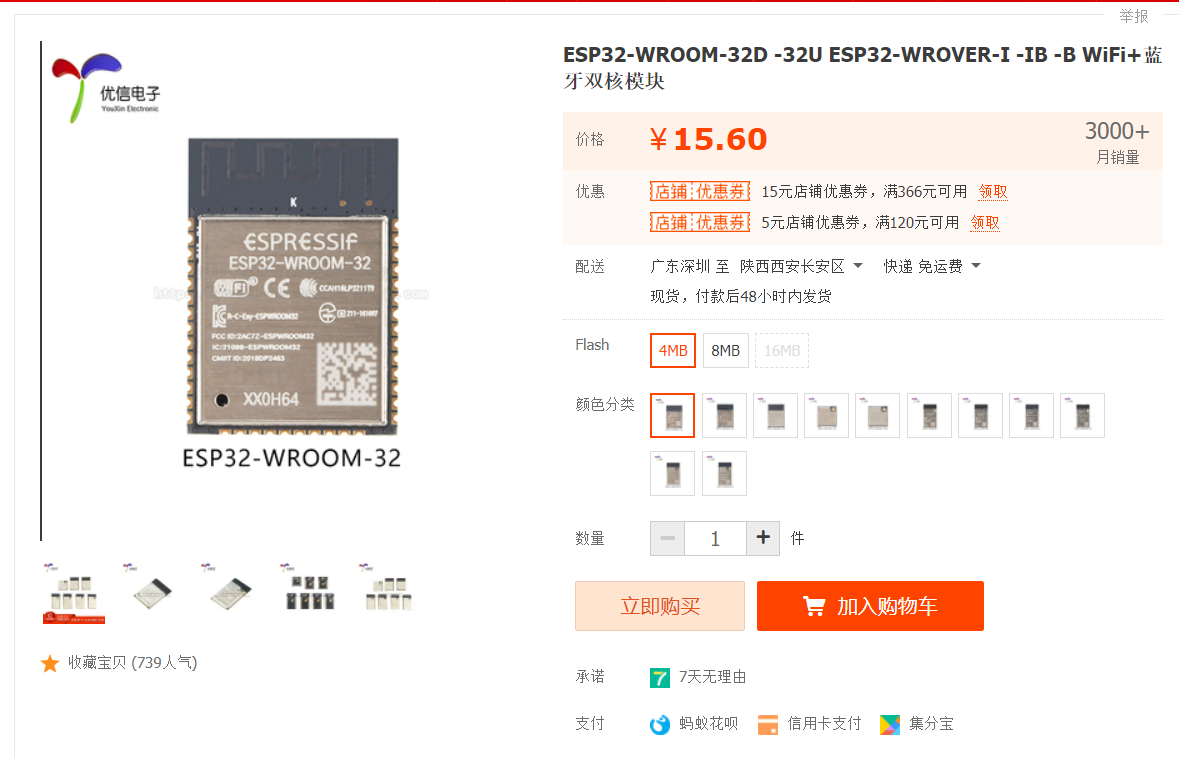### 电源

（然而事实上组装好之后电池仓被挡住了，根本取不出来，但是好在电池比较坚挺，一直坚持到了调参成功）。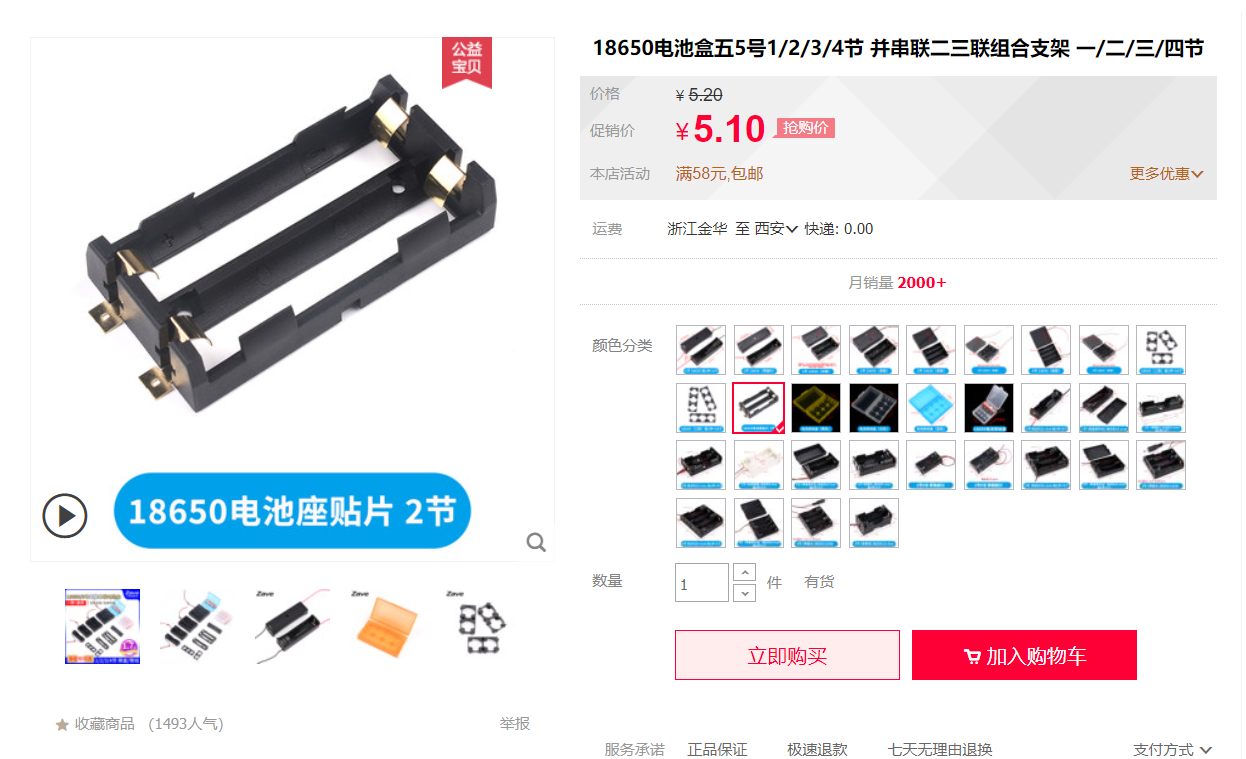### 亚克力底板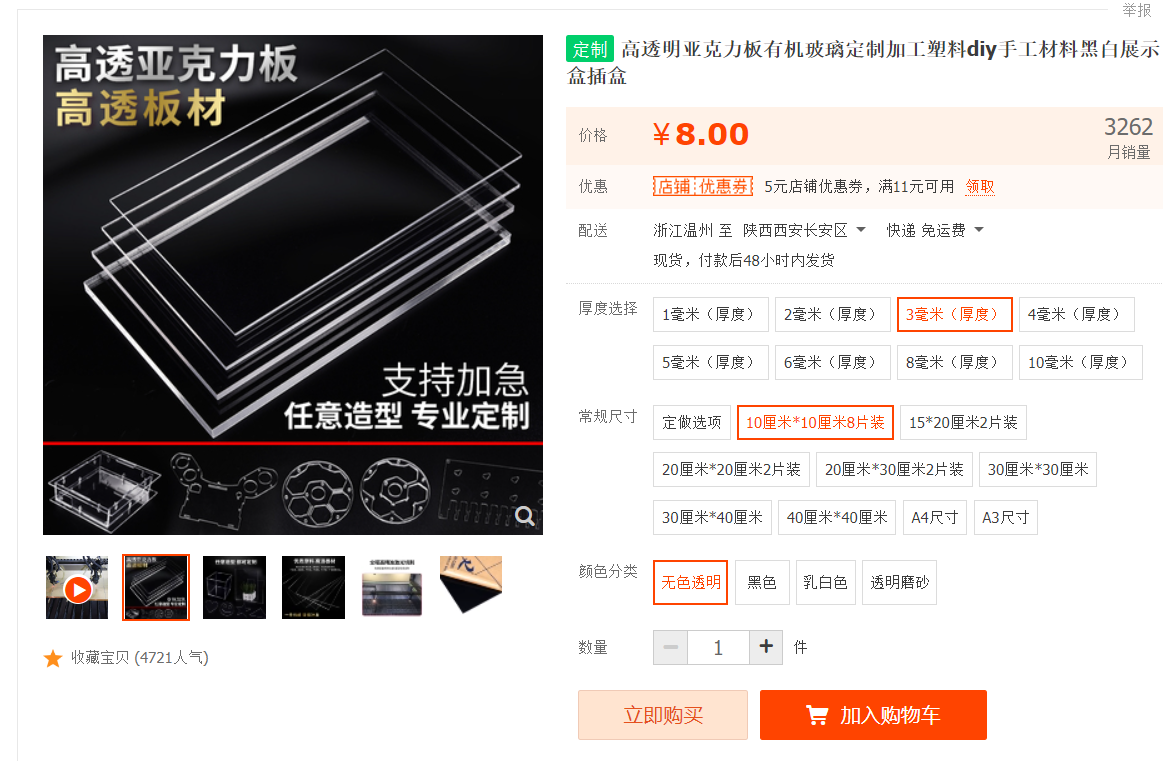## 控制板PCB

### 原理图绘制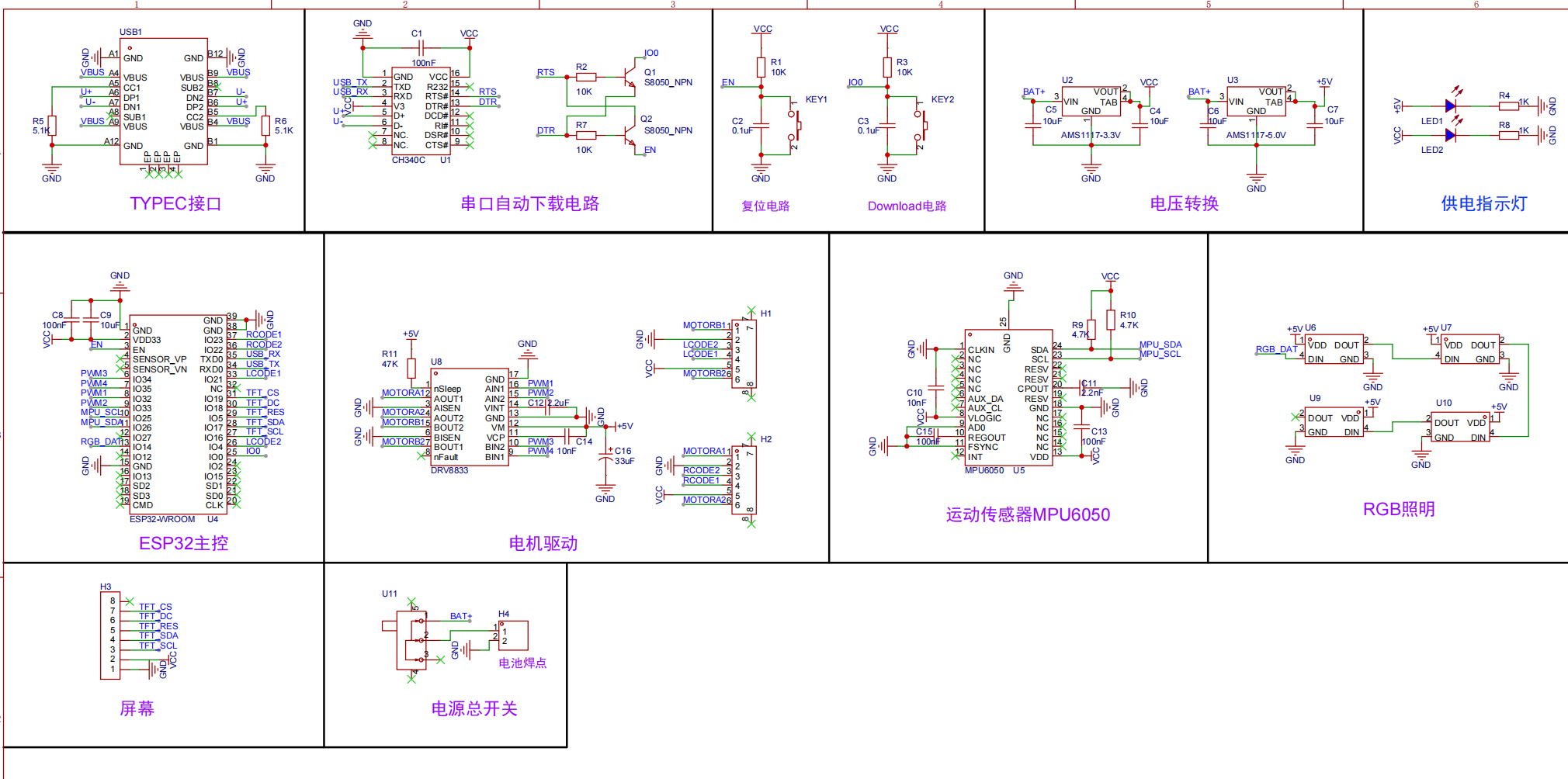### PCB绘制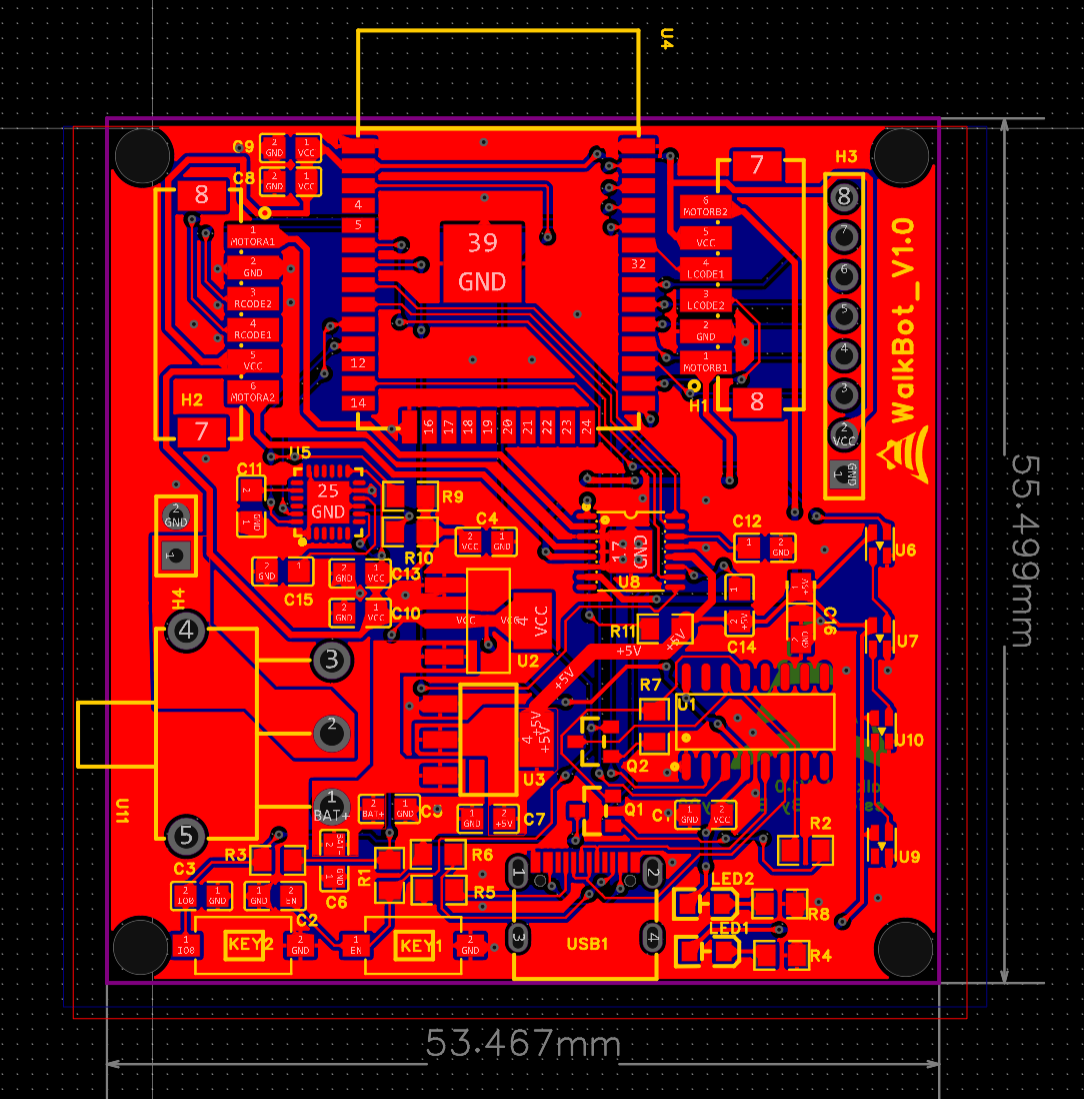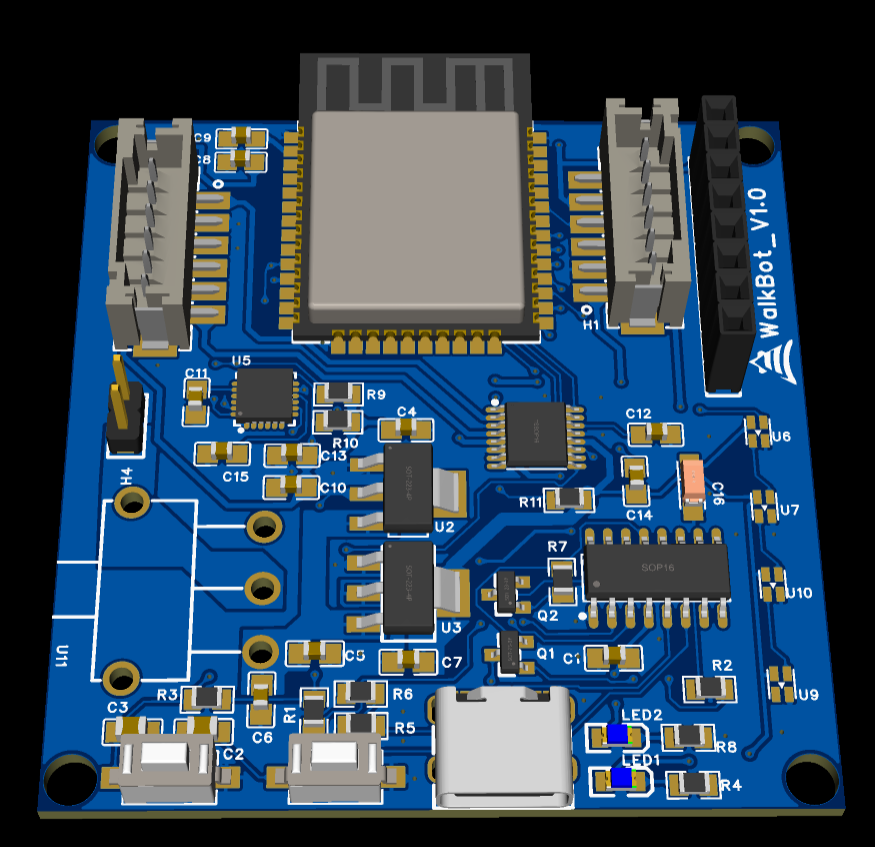## 焊接与组装

• 抹锡膏的时候均匀涂抹，热风枪先对着要焊接的部分整体预热，后均匀加热。
• 检查有没有肉眼可见的连锡，用烙铁加助焊剂把ESP32，CH340C等密脚芯片的各个脚拖焊一遍。
• VCC和GND用万用表连通挡测一下有没有短路。
• 上电时第一时间观察电源指示灯的情况，发现不对立马断开电源。
• 板子上是由焊盘通过硅胶线连接到电池正负极，有短路和线材被扯断的风险，在电池正负极焊盘之间附近打上热熔胶。

• 把电路板放在亚克力中部，对正，平齐，用记号笔画出定位孔。
• 用冲击钻将亚克力板钻出孔位，给电路板的四角装上铜柱，安装到亚克力板上。
• 预估好电机位置，画好定位线，用强力胶水将电机沾到亚克力板的底面侧边，编码器接口朝向地面（这样就无需切割和打孔，直接固定好了电机）。
• 电机的线从底面的同一侧引出，方便后面接到板子上。
• 电池仓贴到亚力克板上，用双面胶固定

1. 接线端子封装画错了

1. MPU6050读不出数据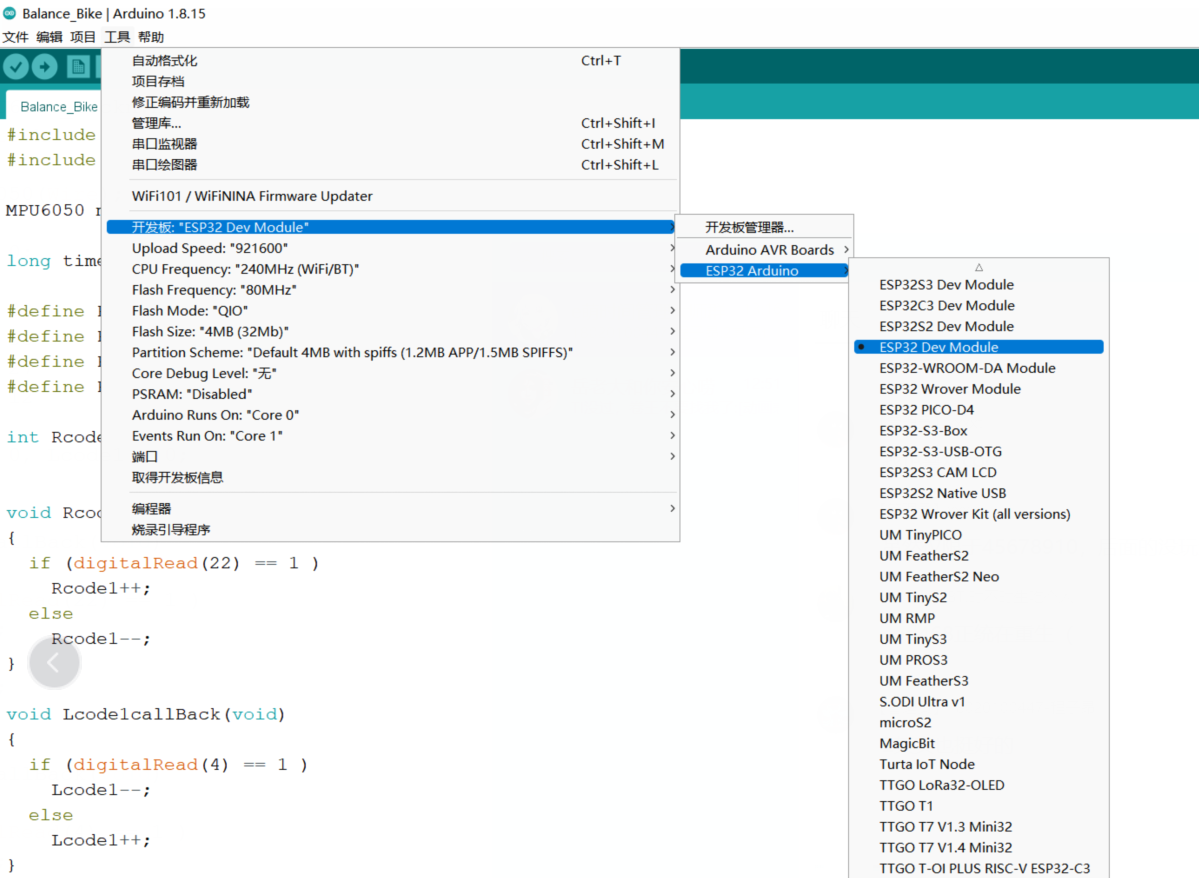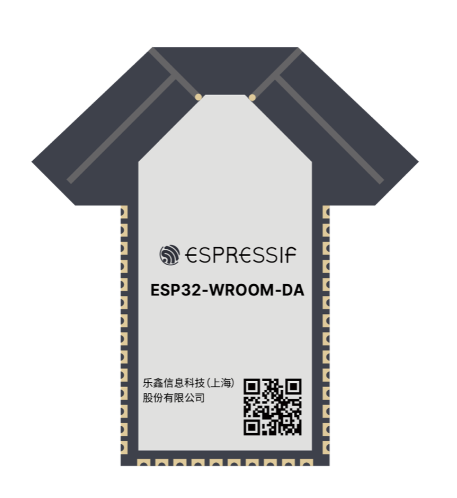1. 电机只有一个转
测试电机的时候发现一边转，另一边不转，说明电机驱动芯片功能肯定是好的。问题要么是虚焊，要么是PWM没有输出。
用联通挡一测，发现没有虚焊。
用万用表的电压档测，发现不转的那一侧的IO34和IO35的PWM没有输出。
搜索引擎查阅资料，发现是ESP32的管脚功能问题，ESP32比较多管脚的系列的的IO34-39只能用作输入，不能用作输出。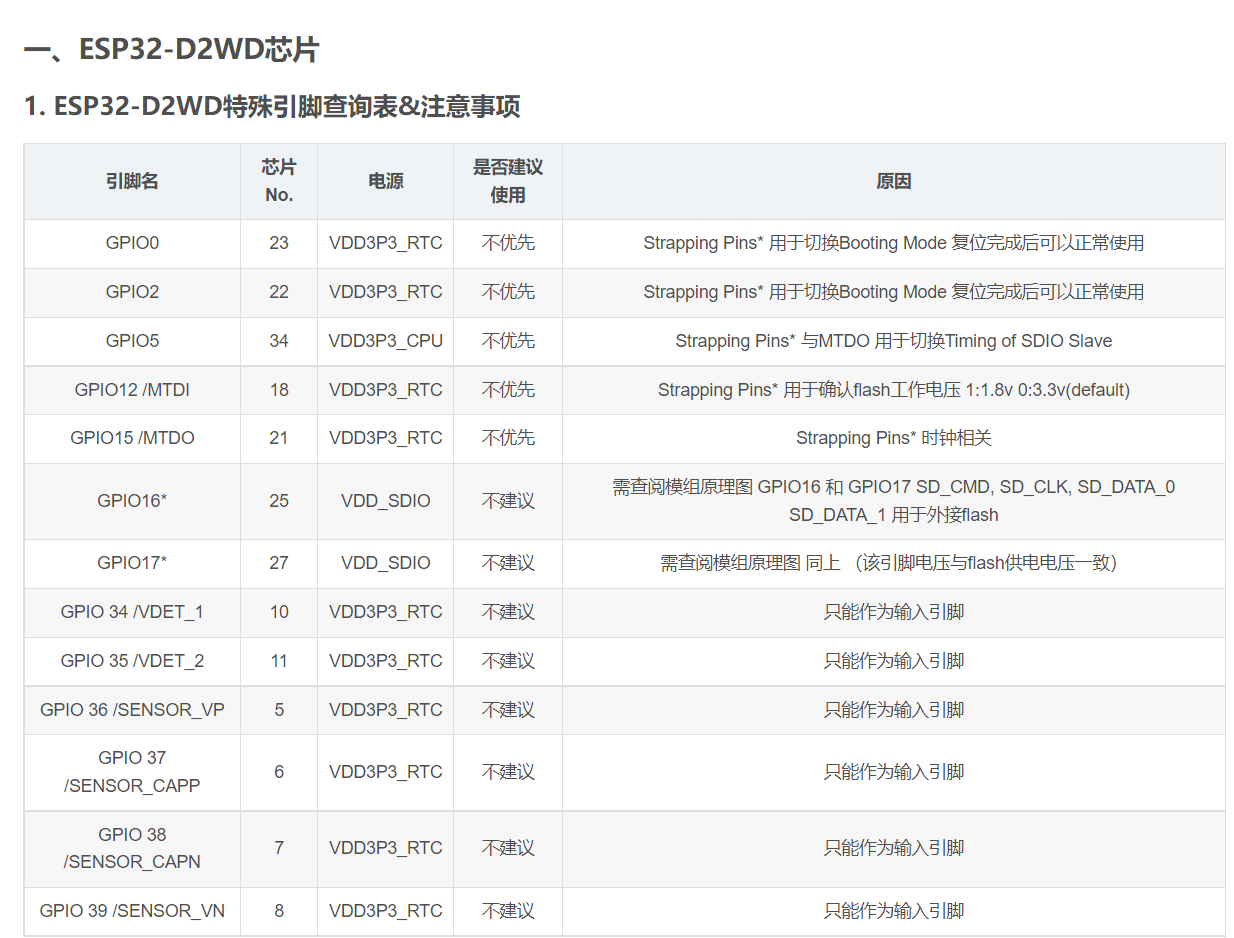于是我直接把IO34和IO35就近飞线到最近的能用的管脚IO12和IO27上，然后在代码中改一下PWM的输出脚，电机就能转动了。

## 代码编写

• MPU6050和电机驱动没啥好讲的，都是现成的库，下载来直接用就可以。
• PWM使用的是ledc函数，调节占空比。
• 可以用串口命令发送PID参数，避免了改一下就要重新烧录的问题

### 调PID

PID深度解析（基于STM32平衡小车）_Carbon6的博客-CSDN博客_平衡车控制算法

Balance_Bike.zip

## 一些照片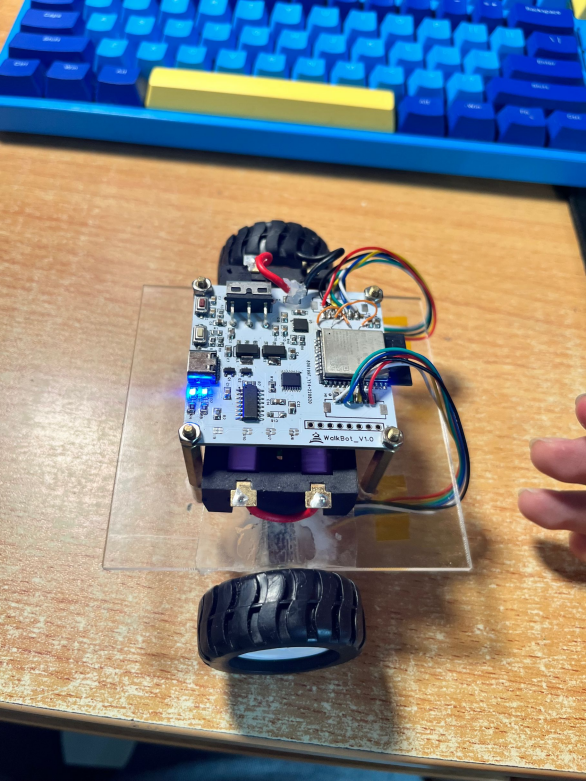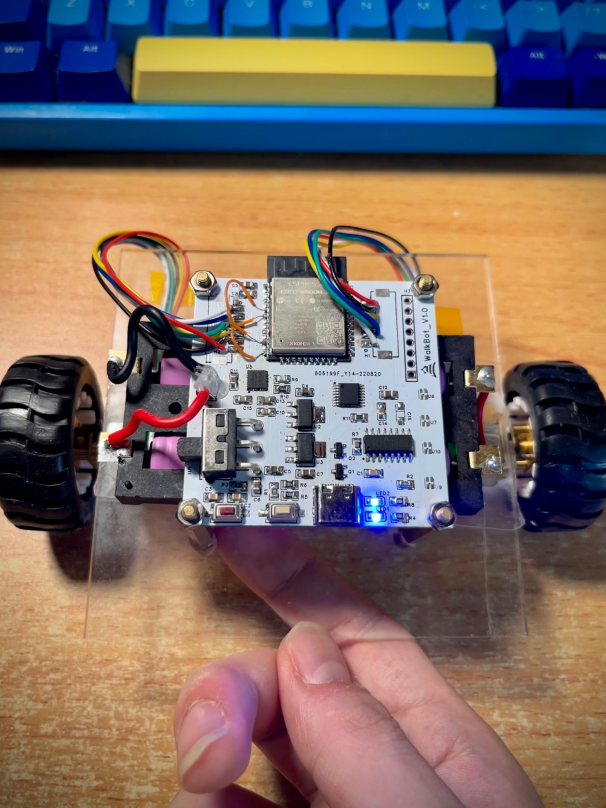## 源代码

#include "MPU6050_tockn.h"
#include "Wire.h"

MPU6050 mpu6050(Wire);

long timer = 0;

#define PWM1 32
#define PWM2 33
#define PWM3 27
#define PWM4 12

int Rcode1 = 0, Lcode1 = 0;

void Rcode1callBack(void)
{
if (digitalRead(22) == 1 )
Rcode1++;
else
Rcode1--;
}

void Lcode1callBack(void)
{
if (digitalRead(4) == 1 )
Lcode1--;
else
Lcode1++;
}

void Motor_Left_Setspeed(int Motorspeed)
{
if (Motorspeed > 0)
{
int PWM = Motorspeed ;  // 正转
if (PWM > 1000)
PWM = 1000;
ledcWrite(0, 0);                     // 设置占空比
ledcWrite(1, PWM);
}
else
{
int PWM = (-Motorspeed) ;  // 反转
if (PWM > 1000)
PWM = 1000;
ledcWrite(0, PWM);                     // 设置占空比
ledcWrite(1, 0);
}
}

void Motor_Right_Setspeed(int Motorspeed)
{
if (Motorspeed > 0)
{
int PWM = Motorspeed ;  // 正转
if (PWM > 1000)
PWM = 1000;
ledcWrite(2, PWM);    // 设置占空比
ledcWrite(3, 0);
}
else
{
int PWM = (-Motorspeed) ;  // 反转
if (PWM > 1000)
PWM = 1000;
ledcWrite(2, 0);     // 设置占空比
ledcWrite(3, PWM);
}
}

int Mechanical_balance = -3;
int balance_UP_KP = 100;
int balance_UP_KD = 8;
/*******************************************************************
函数功能：直立PD控制
入口参数：角度、角速度
返回  值：直立控制PWM
******************************************************************/
int balance_UP(float Angle, float Gyro)
{
float Bias;    //  角度误差
int balance;   //  直立环计算出来的电机转速PWM值
Bias = Angle - Mechanical_balance;
// 求出平衡的角度中值和机械相关
balance = balance_UP_KP * Bias + balance_UP_KD * Gyro;
//===计算平衡控制的电机PWM  PD控制   KP是P系数 KD是D系数
return balance;
}

/**************************************
函数功能：速度PI控制
入口参数：电机编码器的值
返回值：速度控制PWM
**************************************/
int velocity_KP = 2;
float velocity_KI = 0.01;
int delaytime = 5;
int velocity(int encoder_left, int encoder_right)
{
static float Velocity, Encoder_Least, Encoder, Movement;
static float Encoder_Integral;
//=============速度PI控制器=======================//
Encoder_Least = (encoder_left + encoder_right) - 0;    //===获取最新速度偏差    测量速度（左右编码器之和）-目标速度（此处为零）

Encoder *= 0.7;
Encoder += Encoder_Least * 0.3; //  低通滤波

Encoder_Integral += Encoder;  //   积分出位移 积分时间：delaytime

if (Encoder_Integral > 10000)    Encoder_Integral = 10000;   // 积分限幅
if (Encoder_Integral < -10000)    Encoder_Integral = -10000; // 积分限幅

Velocity = Encoder * velocity_KP + Encoder_Integral * velocity_KI; // 速度控制

//  if (pitch < -40 || pitch > 40)   Encoder_Integral = 0;  //===电机关闭后清除积分

return Velocity;
}

int Straight_KS = 20;
/**************************************
函数功能：两侧电机同速控制
入口参数：电机编码器的值
返回值：电机同转速控制PWM
**************************************/
int Straight_compensation(int encoder_left, int encoder_right)
{
int diff = encoder_left - encoder_right;
int compensation = Straight_KS * diff;
return compensation;
}

void setup()
{
Serial.begin(115200);
ledcSetup(0, 10000, 10);  //设置LEDC通道0频率为10K，分辨率为10位，即占空比可选0~1023
ledcSetup(1, 10000, 10);
ledcSetup(2, 10000, 10);
ledcSetup(3, 10000, 10);

ledcAttachPin(PWM1, 0);     //设置LEDC通道0在IO1上输出
ledcAttachPin(PWM2, 1);
ledcAttachPin(PWM3, 2);
ledcAttachPin(PWM4, 3);

ledcWrite(0, 0);        // 设置占空比
ledcWrite(1, 0);
ledcWrite(2, 0);
ledcWrite(3, 0);

pinMode(23 , INPUT_PULLDOWN); // Rcode1
pinMode(22 , INPUT_PULLDOWN); // Rcode2
pinMode(21 , INPUT_PULLDOWN); // Lcode1
pinMode(4 ,  INPUT_PULLDOWN);  // Lcode2

attachInterrupt(23, Rcode1callBack, RISING);  //当电平上升沿时，触发中断
attachInterrupt(21, Lcode1callBack, RISING);

Wire.begin(26, 25);
mpu6050.begin();
mpu6050.calcGyroOffsets(true);

}

int n = 0;
void loop()
{
delay(delaytime);
mpu6050.update();

int balance_PWM = balance_UP(mpu6050.getAngleY(), mpu6050.getGyroY());
int velocity_PWM = velocity(Lcode1, Rcode1);
int Final_PWM = balance_PWM - velocity_PWM;
int compensation_PWM = Straight_compensation(Lcode1, Rcode1);
Motor_Left_Setspeed(Final_PWM);
Motor_Right_Setspeed(Final_PWM + compensation_PWM);

n++;
if (n == 50)
{
Serial.println("===================");
Serial.print("tgyroY : "); Serial.print(mpu6050.getGyroY());      // 打印角度
Serial.print("\t angleY : "); Serial.println(mpu6050.getAngleY());
Serial.print("Lcode1 = ");  Serial.print(Lcode1);                 // 打印编码器计数
Serial.print("Rcode1 = ");  Serial.println(Rcode1);
Serial.print("balancePWM = ");  Serial.println(balance_PWM);      // 打印直立环PWM值
Serial.print("velocityPWM = ");  Serial.println(velocity_PWM);    // 打印速度环PWM值
Serial.print("compensationPWM = ");  Serial.println(compensation_PWM);    // 打印同速环PWM值
Serial.println("==================\n");
n = 0;
}

Rcode1 = 0, Lcode1 = 0; // 清空计数

if (Serial.available() > 0)//判读是否串口有数据
{
String comdata = "";//缓存清零
while (Serial.available() > 0)//循环串口是否有数据
{
comdata += char(Serial.read()); //叠加数据到comdata
delay(2);//延时等待
}
if (comdata.indexOf('p') != -1)
{
String substr = comdata.substring(2); // 从第三位开始截取
balance_UP_KP = substr.toInt(); // 转成整数
}
else if (comdata.indexOf('d') != -1)
{
String substr = comdata.substring(2);  // 从第三位开始截取
balance_UP_KD = substr.toInt(); // 转成整数
}
else if (comdata.indexOf('v') != -1)
{
String substr = comdata.substring(2);  // 从第三位开始截取
velocity_KP = substr.toInt(); // 转成整数
}
else if (comdata.indexOf('i') != -1)
{
String substr = comdata.substring(2); // 从第三位开始截取
velocity_KI = substr.toFloat(); // 转成整数
}
else if (comdata.indexOf('s') != -1)
{
String substr = comdata.substring(2); // 从第三位开始截取
Straight_KS = substr.toFloat(); // 转成整数
}
else if (comdata.indexOf('m') != -1)
{
String substr = comdata.substring(2);  // 从第三位开始截取
Mechanical_balance = substr.toInt(); // 转成整数
}
else if (comdata.indexOf('t') != -1)
{
String substr = comdata.substring(2);  // 从第三位开始截取
delaytime = substr.toInt(); // 转成整数
}
}

}

目录